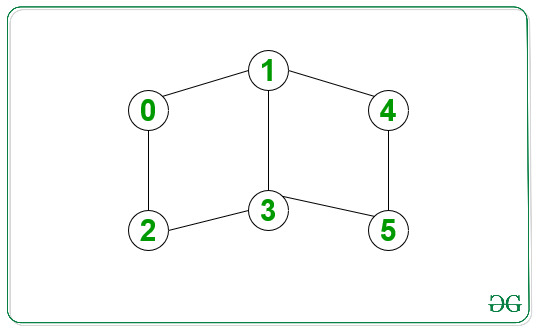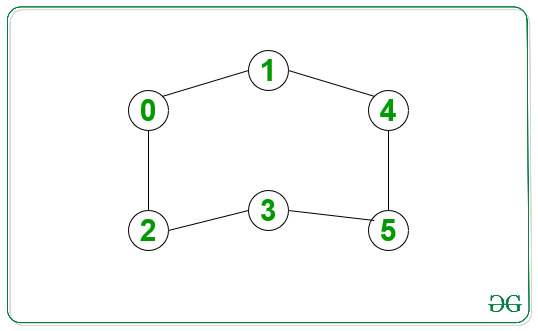# Print all shortest paths between given source and destination in an undirected graph

Given an undirected and unweighted graph and two nodes as source and destination, the task is to print all the paths of the shortest length between the given source and destination.

Examples:

Input: source = 0, destination = 5Output:
0 -> 1 -> 3 -> 5
0 -> 2 -> 3 -> 5
0 -> 1 -> 4 -> 5
Explanation:
All the above paths are of length 3, which is the shortest distance between 0 and 5.

Input: source = 0, destination = 4Output:
0 -> 1 -> 4

## Recommended: Please try your approach on {IDE} first, before moving on to the solution.

Approach: The is to do a Breadth First Traversal (BFS) for a graph. Below are the steps:

1. Start BFS traversal from source vertex.
2. While doing BFS, store the shortest distance to each of the other nodes and also maintain a parent vector for each of the nodes.
3. Make the parent of source node as “-1”. For each node, it will store all the parents for which it has the shortest distance from the source node.
4. Recover all the paths using parent array. At any instant, we will push one vertex in the path array and then call for all its parents.
5. If we encounter “-1” in the above steps, then it means a path has been found and can be stored in the paths array.

Below is the implementation of the above approach:

 `// Cpp program for the above approach ` `#include ` `using` `namespace` `std; ` ` `  `// Function to form edge between ` `// two vertices src and dest ` `void` `add_edge(vector<``int``> adj[], ` `              ``int` `src, ``int` `dest) ` `{ ` `    ``adj[src].push_back(dest); ` `    ``adj[dest].push_back(src); ` `} ` ` `  `// Function which finds all the paths ` `// and stores it in paths array ` `void` `find_paths(vector >& paths, ` `                ``vector<``int``>& path, ` `                ``vector<``int``> parent[], ` `                ``int` `n, ``int` `u) ` `{ ` `    ``// Base Case ` `    ``if` `(u == -1) { ` `        ``paths.push_back(path); ` `        ``return``; ` `    ``} ` ` `  `    ``// Loop for all the parents ` `    ``// of the given vertex ` `    ``for` `(``int` `par : parent[u]) { ` ` `  `        ``// Insert the current ` `        ``// vertex in path ` `        ``path.push_back(u); ` ` `  `        ``// Recursive call for its parent ` `        ``find_paths(paths, path, parent, ` `                   ``n, par); ` ` `  `        ``// Remove the current vertex ` `        ``path.pop_back(); ` `    ``} ` `} ` ` `  `// Function which performs bfs ` `// from the given souce vertex ` `void` `bfs(vector<``int``> adj[], ` `         ``vector<``int``> parent[], ` `         ``int` `n, ``int` `start) ` `{ ` `    ``// dist will contain shortest distance ` `    ``// from start to every other vertex ` `    ``vector<``int``> dist(n, INT_MAX); ` ` `  `    ``queue<``int``> q; ` ` `  `    ``// Insert source vertex in queue and make ` `    ``// its parent -1 and distance 0 ` `    ``q.push(start); ` `    ``parent[start] = { -1 }; ` `    ``dist[start] = 0; ` ` `  `    ``// Untill Queue is empty ` `    ``while` `(!q.empty()) { ` `        ``int` `u = q.front(); ` `        ``q.pop(); ` `        ``for` `(``int` `v : adj[u]) { ` `            ``if` `(dist[v] > dist[u] + 1) { ` ` `  `                ``// A shorter distance is found ` `                ``// So erase all the previous parents ` `                ``// and insert new parent u in parent[v] ` `                ``dist[v] = dist[u] + 1; ` `                ``q.push(v); ` `                ``parent[v].clear(); ` `                ``parent[v].push_back(u); ` `            ``} ` `            ``else` `if` `(dist[v] == dist[u] + 1) { ` ` `  `                ``// Another candidate parent for ` `                ``// shortes path found ` `                ``parent[v].push_back(u); ` `            ``} ` `        ``} ` `    ``} ` `} ` ` `  `// Function which prints all the paths ` `// from start to end ` `void` `print_paths(vector<``int``> adj[], ` `                 ``int` `n, ``int` `start, ``int` `end) ` `{ ` `    ``vector > paths; ` `    ``vector<``int``> path; ` `    ``vector<``int``> parent[n]; ` ` `  `    ``// Function call to bfs ` `    ``bfs(adj, parent, n, start); ` ` `  `    ``// Function call to find_paths ` `    ``find_paths(paths, path, parent, n, end); ` ` `  `    ``for` `(``auto` `v : paths) { ` ` `  `        ``// Since paths contain each ` `        ``// path in reverse order, ` `        ``// so reverse it ` `        ``reverse(v.begin(), v.end()); ` ` `  `        ``// Print node for the current path ` `        ``for` `(``int` `u : v) ` `            ``cout << u << ``" "``; ` `        ``cout << endl; ` `    ``} ` `} ` ` `  `// Driver Code ` `int` `main() ` `{ ` `    ``// Number of vertices ` `    ``int` `n = 6; ` ` `  `    ``// array of vectors is used ` `    ``// to store the graph ` `    ``// in the form of an adjacency list ` `    ``vector<``int``> adj[n]; ` ` `  `    ``// Given Graph ` `    ``add_edge(adj, 0, 1); ` `    ``add_edge(adj, 0, 2); ` `    ``add_edge(adj, 1, 3); ` `    ``add_edge(adj, 1, 4); ` `    ``add_edge(adj, 2, 3); ` `    ``add_edge(adj, 3, 5); ` `    ``add_edge(adj, 4, 5); ` ` `  `    ``// Given source and destination ` `    ``int` `src = 0; ` `    ``int` `dest = n - 1; ` ` `  `    ``// Function Call ` `    ``print_paths(adj, n, src, dest); ` ` `  `    ``return` `0; ` `} `

Output:

```0 1 3 5
0 2 3 5
0 1 4 5
```

Time Complexity: O(V + E) where V is the number of vertices and E is the number of edges.
Auxiliary Space: O(V) where V is the number of vertices.My Personal Notes arrow_drop_upCheck out this Author's contributed articles.

If you like GeeksforGeeks and would like to contribute, you can also write an article using contribute.geeksforgeeks.org or mail your article to contribute@geeksforgeeks.org. See your article appearing on the GeeksforGeeks main page and help other Geeks.

Please Improve this article if you find anything incorrect by clicking on the "Improve Article" button below.